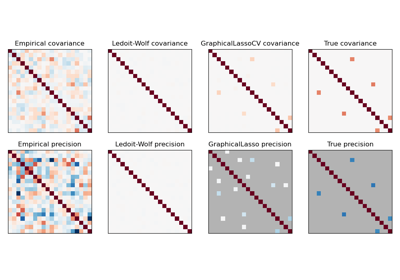# sklearn.covariance.ledoit_wolf¶

sklearn.covariance.ledoit_wolf(X, *, assume_centered=False, block_size=1000)[source]

Estimates the shrunk Ledoit-Wolf covariance matrix.

Read more in the User Guide.

Parameters
Xarray-like of shape (n_samples, n_features)

Data from which to compute the covariance estimate

assume_centeredbool, default=False

If True, data will not be centered before computation. Useful to work with data whose mean is significantly equal to zero but is not exactly zero. If False, data will be centered before computation.

block_sizeint, default=1000

Size of the blocks into which the covariance matrix will be split. This is purely a memory optimization and does not affect results.

Returns
shrunk_covndarray of shape (n_features, n_features)

Shrunk covariance.

shrinkagefloat

Coefficient in the convex combination used for the computation of the shrunk estimate.

Notes

The regularized (shrunk) covariance is:

(1 - shrinkage) * cov + shrinkage * mu * np.identity(n_features)

where mu = trace(cov) / n_features

## Examples using sklearn.covariance.ledoit_wolf¶